# Teacher-Tested Tips and Activities for Teaching the Area Model Multiplication Method

This method provides a lot more than just the answer to the equation.

When you were in school, you probably learned to multiply large numbers using the standard algorithm method . Students still learn that method today, but they also learn a variety of other options to help them truly understand the process. One of these is area model multiplication, which seems more complicated at first but actually really benefits kids. Let’s take a look at what this method is, why it matters, and how to teach it.

## What is area model multiplication?

The area model method is based on the simple equation used to find the area of a rectangle: the length times the width equals the total area (LxW=A). Students usually start by learning simple arrays for single-digit multiplication, demonstrated by this anchor chart from Primary Punch.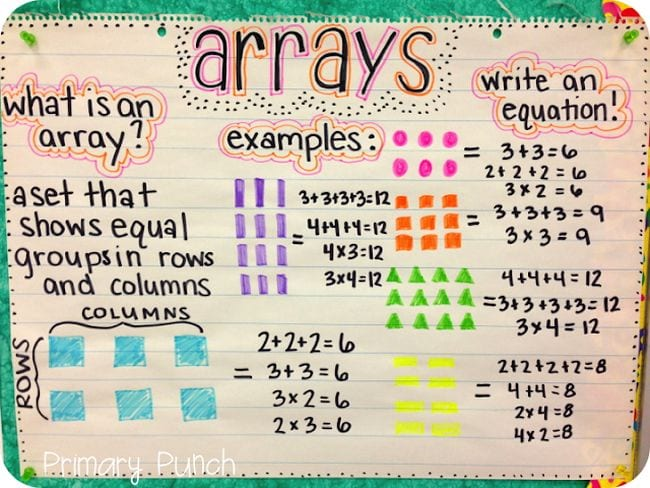As students proceed to more complicated equations, the area model becomes more complex. Breaking equations down by place value, students multiply and add to reach an answer.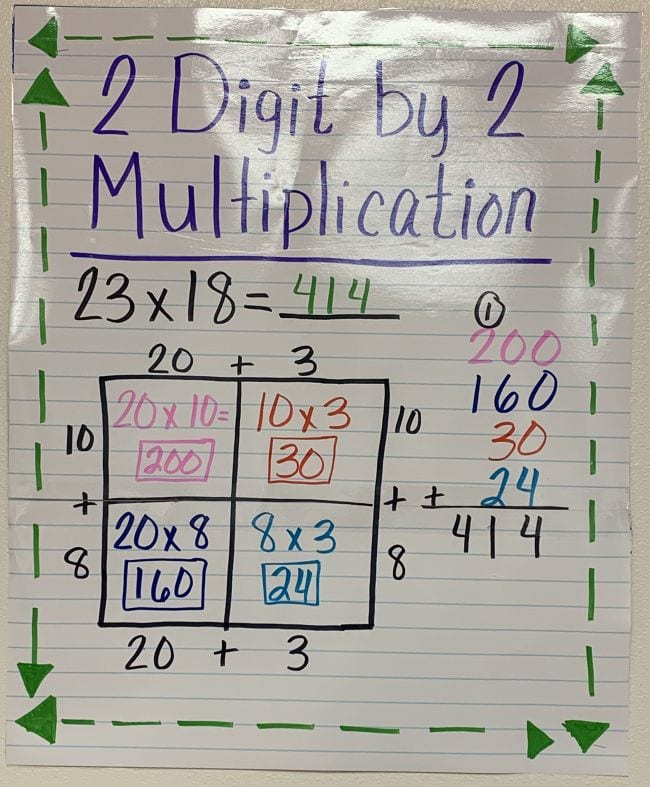Amanda Gonzalez/Pinterest

As students work with higher numbers, the box model expands to include each place value. This multiplication method can be used with fractions, decimals, and algebraic equations too.

[contextly_auto_sidebar]

## Why do we teach the area model?

Many people’s first thought on seeing the area model method laid out is, “Oh, that seems so complicated! Why can’t they just do it the old-fashioned way like I did growing up?” Turns out, there are some very good answers to that question. Area model multiplication gives students another way to visualize a math equation, which is extremely valuable. Not all kids (or adults) think in the same way. When you give them more ways to approach a math problem, they’re more likely to succeed.

More importantly, the area model method helps students understand how math works. And as the equations they solve become more complicated, the purpose behind area models becomes much more clear. Lauren of Leaf and STEM Learning puts it this way:

Methods like area models are developed with the purpose of gaining a lasting understanding of the mechanics of math rather than simply the answer to a quick math problem. The standard algorithm is often the most efficient way to solve a problem, but it often hides the reasoning of the math from students learning to do more complicated work at younger and younger ages. Yes, the area model looks very different from the math many of us did as children, but the mechanics are the same.

On a WeAreTeachers HELPLINE discussion , Nico O. shared an example that shows that while the area model may seem overly-complicated for simple problems, it actually becomes an easier method (and one that avoids mistakes) for more complicated equations.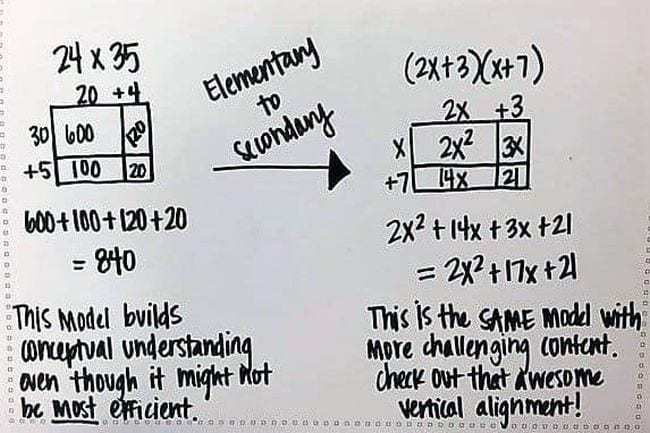## Area Model Multiplication Activities

Once students get the hang of area method multiplication, they can use it as a primary problem-solving method or a way to check their answers. Here are some activities for introducing this method and using it at a variety of levels.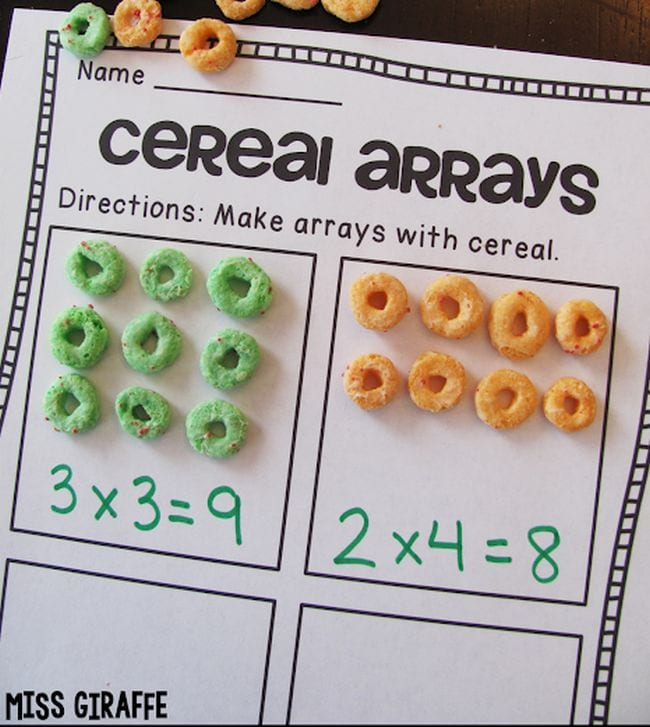Multiplication arrays are the basis for the area model method. We love the idea of using cereal to demonstrate arrays (and have a snack too). Visit the link for more array ideas from Miss Giraffe.

## 2. Lay arrays on the multiplication table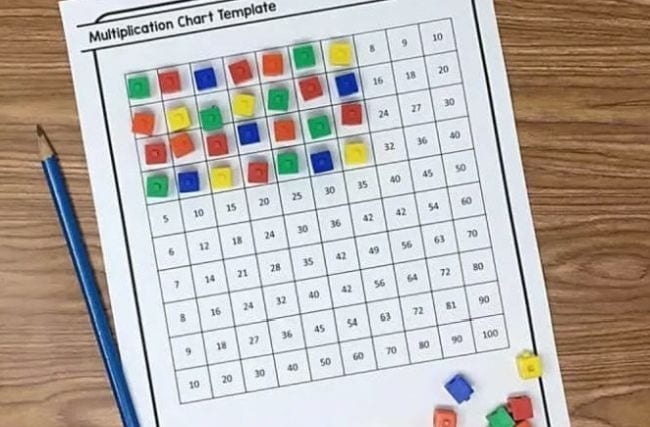Help students see the connection between arrays and multiplication facts by laying arrays over the multiplication table itself. The lower right corner will always match the number of blocks they’ve laid out.

## 3. Help parents understand how area model multiplication works

Sometimes parents say they’ve given up on so-called “new math.” It’s so different from the way they learned that they can’t even help their kids with their homework. Try sharing this video with them so they can see the area method in action and help their kids as needed.

## 4. Sing the Area Model Multiplication SongTeacher Rayli M. recommended this video. “I played this song for my students, and they liked it!” She does warn that it’s likely to get stuck in your head, so beware!

## 5. Use base 10 blocks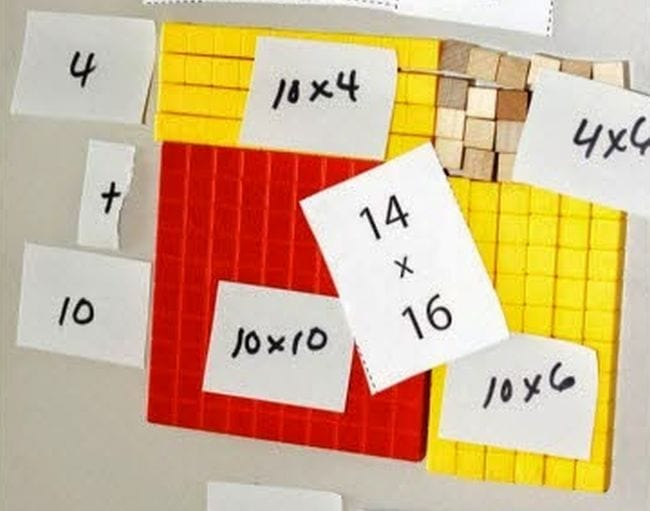Base 10 blocks are perfect for getting some hands-on practice with the area method. Use sticky notes to mark the total for each section as you work. (Teaching online? Use these free digital base 10 blocks instead.)

## 6. Introduce the box model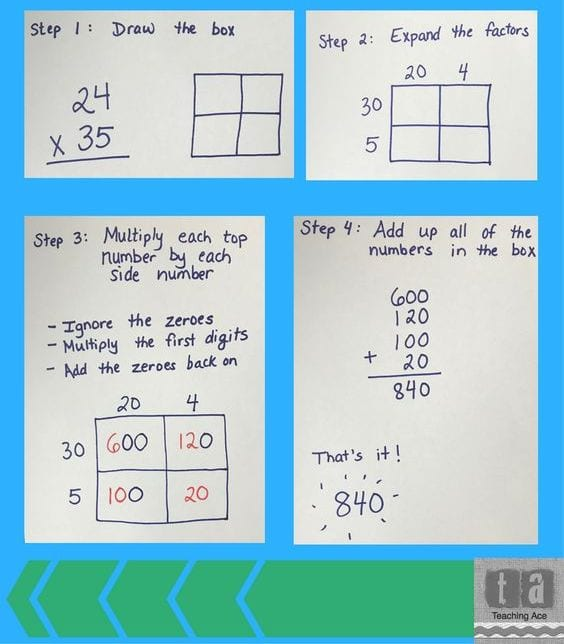Now students are ready to lay the equation out in a box model. In the HELPLINE discussion, Melissa S. recommends using different colors for each place value (let kids pick the colors to add a little fun). “It helps them see the expanded form more. I also teach them to multiply the first numbers and then count the zeros.” Zara A. suggests having kids work in teams, with each student responsible for completing one of the boxes before they’re all added together.

## 7. Fill in multiplication challenge puzzles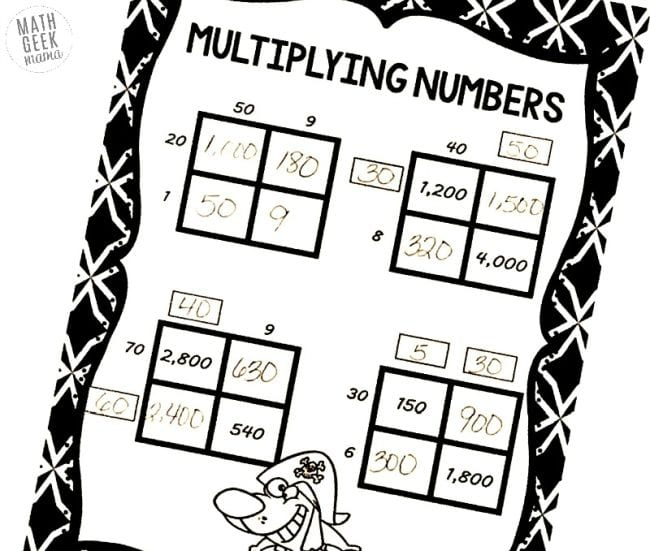These free puzzles help kids practice completing each of the boxes without worrying about the ultimate answer just yet. Some of the puzzles require kids to figure out the place value boxes, too, sneaking in a little division practice!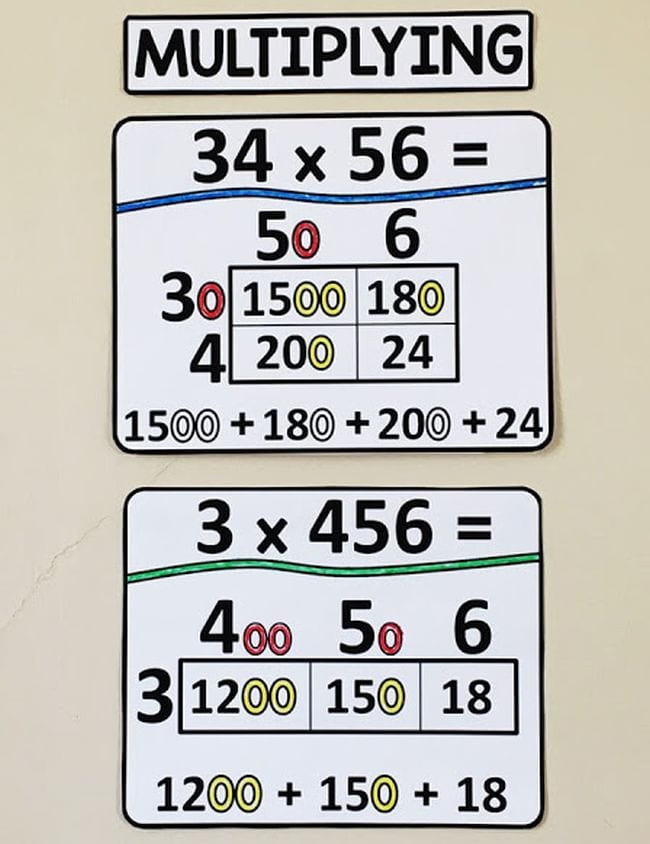Put examples of area method multiplication up on your math word wall. Kids can use them as a reminder when they get stuck on a problem.

## 9. Try it for fractionsArea method is a cool way to visualize how we multiply fractions. Learn how it works and get some free cards to try out at the link.

## 10. Remind kids this is just one possible method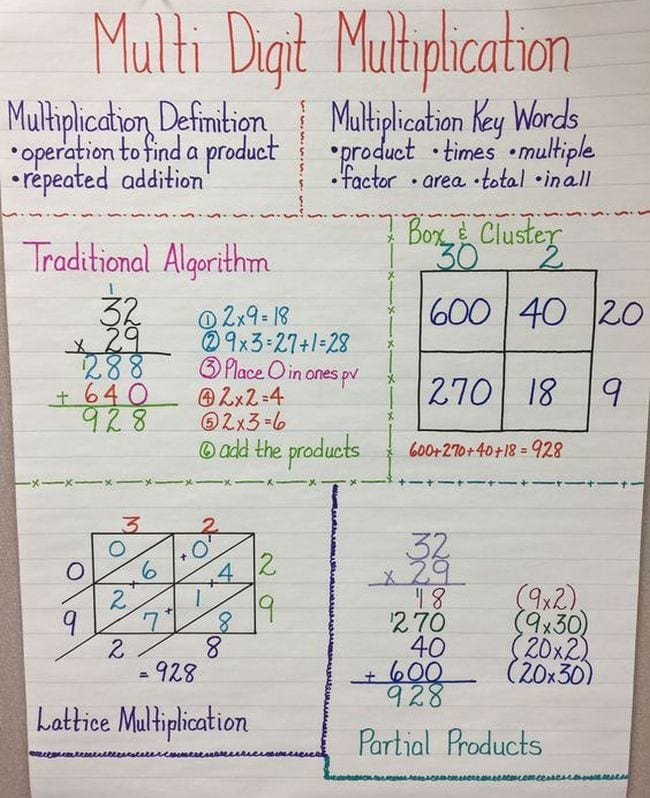There are several ways to approach multiplication, so if kids have trouble with one method, remind them they’re welcome to use other options. Stress that using more than one way to solve a problem helps ensure the correct answer, too.

Looking for more ideas? Check out 30 Fun Hands-On Ways to Teach Multiplication.

Plus, How Saying “Times” When Teaching Multiplication Confuses Students.## You Might Also Like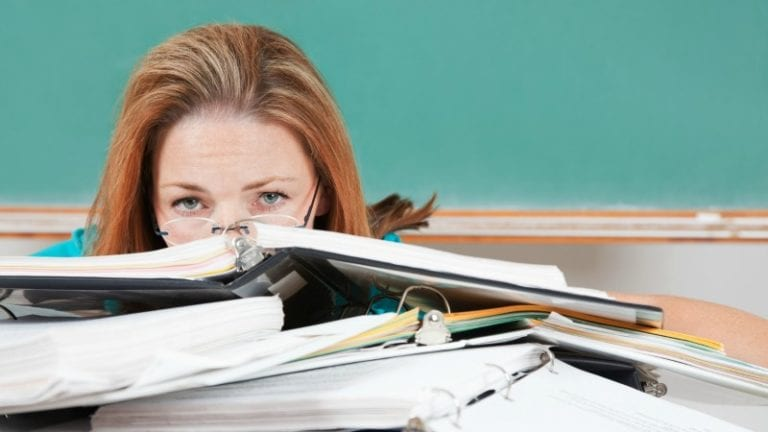### 10 Books to Read When You Feel Stuck in Your Job

C'mon baby, light that fire!